A.1 The Distance Between Words.
To define the "distance" between words, we must first define the distance between an ELS representing a word and a string of letters (SL) in the text, (i.e. with d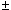1) representing the other word. Before we can do that, we must define the distance between ELS and SL in a given array: and before we can do that, we must define the distance between individual letters in the array.

As indicated in Section 1, we think of an array as one long line that spirals down on a cylinder; its row length h is the number of vertical columns. To define the distance between two letters x and x', cut the cylinder along a vertical line between two columns. In the resulting plane each of x and x' have two integer coordinates. and we compute the distance between them as usual, using these coordinates. In general, there are two possible values for this distance, depending on the vertical line that was chosen for cutting the cylinder; if the two values are different, we use the smaller one.

Next, we define the distance between fixed ELS e and SL e' in a fixed cylindrical array. Set

f := the distance between consecutive letters of e,

f ' := the distance between consecutive letters of e' = 1.

l := the minimal distance between a letter of e and one of e',

and define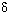(e, e') := f2 + f'2+l2+1. We call(e, e') the distance between the ELS e and the SL e' in the given array; it is small if both fit into a relatively compact area.

Now there are many ways of writing Genesis as a cylindrical array, depending on the row length h. Denote byh(e, e') the distance(e, e') in the array determined by h, and set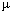h(e, e') := 1/h(e, e'); the largerh(e, e') is, the more compact is the configuration consisting of e and e' in the array with row length h. Set e = (n, d, k) (recall that d is the skip). Of particular interest are the row lengths h = h1, h2,.... where hi is the integer nearest to |d| /i (1/2 is rounded up). Thus when h = h1 = |d|, then e appears as a column of adjacent letters and when h = h2, then e appears either as a column that skips alternate rows or as a straight line of knight's moves. In general, the arrays in which e appears relatively compactly are those with row length hi with i "not too large."

The above discussion indicates that if there is an array in which the configuration (e,e') is unusually compact, it is likely to be among those whose row length is one of the first ten hi. (Here and in the sequel 10 is an arbitrarily selected "moderate" number). So setting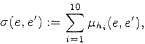we conclude that(e, e') is a reasonable measure of the maximal "compactness" of the configuration (e, e') in any array. Equivalently, it is an inverse measure of the minimum distance between e and e'.

Next, given a word w, we look for the most "noteworthy" occurrence or occurrences of w as an ELS in G. For this, we chose ELS's e = (n,d,k) with |d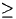2 that spell out w for which |d| is minimal over all of G, or at least over large portions of it. Specifically, define the domain of minimality of e as the maximal segment Te of G that includes e and does not include any other ELS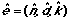for w with |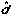|< |d|. The length of Te, relative to the whole of G, is the "weight" we assign to e. Thus we define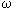(e) :=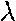(Te)/(G), where(Te) is the length of Te, and(G) is the length of G. For any two words w and w', we set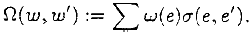where the sum is over all ELS's e spelling out w and over all SL's e' spelling out w'. Roughly,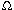(w, w') measures the maximum closeness of the more noteworthy appearances of w as ELS's and w' as SL's in Genesis--the closer they are, the larger is(w, w').

When actually computing(w, w'), the size of the list of ELS's for w may be impractically large (especially for short words). It is clear from the definition of the domain of minimality that ELS's for w with relatively large skips will contribute very little to the value of(w,w') due to their small weight. Hence, in order to cut the amount of computation we restrict beforehand the range of the skip |d|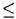D(w) for w so that the expected number of ELS's for w will be 10. This expected number equals the product of the relative frequencies (within Genesis) of the letters constituting w multiplied by the total number of all equidistant letter sequences with 2|d|D. (The latter is given by the formula (D - 1)(2L - (k - 1)(D + 2)), where L is the length of the text and k is the number of letters in w). Abusing our notation somewhat, we continue to denote this modified function by(w,w').

A.2 The Corrected Distance.
In the previous section we defined a measure(w, w') of proximity between two words w and w' -- an inverse measure of the distance between them. We are, however, interested less in the absolute distance between two words, than in whether this distance is larger or smaller than "expected". In this section, we define a "relative distance" c(w, w'), which is small when w is "unusually close" to w', and is 1, or almost 1, when w is "unusually far" from w'.

The idea is to use perturbations of the arithmetic progressions that define the notion of an ELS. Specifically, start by fixing a triple (x,y,z) of integers in the range {-r,...,0,...,r}; there are (2r + 1)3 such triples. In Witztum et al. (1994) and also here we put r = 2. which gives us 125 triples. Next, rather than looking for ordinary ELS's (n,d,k), look for "(x,y,z)-perturbed ELS's" (n,d,k)(x,y,z) obtained by taking the positions

n, n + d,...,n + (k - 4)d, n + (k - 3)d + x, n + (k - 2)d + x + y, n + (k - 1)d + x + y + z,

instead of the positions n, n + d, n +2d,...,n +(k - 1)d. Note that in a word of length k, k-2 intervals could be perturbed. However, we preferred to perturb only the 3 last ones, for technical programming reasons.

The distance between the (x,y,z)-peturbed ELS (n, d, k)(x,y,z) and the SL (n'1,k') is defined by the same formulae as in the non-perturbed case, where f is taken to be the distance between the first two letters of (x,y,z)-perturbed e.

We may now calculate the "(x,y,z)-proximity" of two words w and w' in a manner exactly analogous to that used for calculating the "ordinary" proximity(w, w'). This yields 125 numbers(x,y,z)(w, w'), of which(w,w')=(0,0,0)(w,w') is one. We are interested in only some of these 125 numbers; namely, those corresponding to triples (x,y,z) for which there actually exist some (x,y,z)-perturbed ELS's in Genesis for w (the other(x,y,z)(w,w') vanish). Denote by M(w, w') the set of all such triples, and by m(w, w') the number of its elements.

Suppose (0,0,0) is in M(w, w'), i.e., w actually appears as ordinary ELS (i.e., with x = y = z = 0) in the text. Denote by v(w,w') the number of triples (x,y,z) in M(w,w') for which(x,y,z)(w,w')(w,w'). If m(w,w')10 (again, 10 is an arbitrarily selected "moderate" number),

c(w,w') :=v(w,w')/m(w,w').

If (0, 0,0) is not in M(w, w'), or if m(w, w') < 10 (in which case we consider the accuracy of the method as insufficient), we do not define c(w,w').

In words, the corrected distance c(w,w') is simply the rank order of the proximity(w,w') among all the "perturbed proximities"(x,y,z)(w,w'); if(w,w') is tied with other(x,y,z)(w,w'), half of these others are considered to "exceed"(w,w'). We normalize it so that the maximum distance is 1. A large corrected distance means that ELS's representing w are far away from the SL's representing w', on a scale determined by how far the perturbed ELS's for w are from the SL's for w'.